Thank you for visiting nature.com. You are using a browser version with limited support for CSS. To obtain the best experience, we recommend you use a more up to date browser (or turn off compatibility mode in Internet Explorer). In the meantime, to ensure continued support, we are displaying the site without styles and JavaScript.

# Nonlinearly-enhanced energy transport in many dimensional quantum chaos

## Abstract

By employing a nonlinear quantum kicked rotor model, we investigate the transport of energy in multidimensional quantum chaos. This problem has profound implications in many fields of science ranging from Anderson localization to time reversal of classical and quantum waves. We begin our analysis with a series of parallel numerical simulations, whose results show an unexpected and anomalous behavior. We tackle the problem by a fully analytical approach characterized by Lie groups and solitons theory, demonstrating the existence of a universal, nonlinearly-enhanced diffusion of the energy in the system, which is entirely sustained by soliton waves. Numerical simulations, performed with different models, show a perfect agreement with universal predictions. A realistic experiment is discussed in two dimensional dipolar Bose-Einstein-Condensates (BEC). Besides the obvious implications at the fundamental level, our results show that solitons can form the building block for the realization of new systems for the enhanced transport of matter.

## Introduction

Anderson localization is a fundamental concept that, originally introduced in solid-state physics to describe conduction-insulator transitions in disordered crystals, has permeated several research areas and has become the subject of great research interest1,2,3,4,5,6,7,8,9,10,11. Theories and subsequent experiments demonstrated that disorder favors the formation of spatially localized states, which sustain diffusion breakdown and exponentially attenuated transmission in random media1. Although many properties of wave localization are now well understood, several fundamental questions remains. Perhaps one of the most intriguing problem is related to the transport of energy. Intuitively, one can expect that disorder –by favoring exponentially localized stated– arrests in general any propagation inside a noncrystalline medium. However, the interplay between localization and disorder is nontrivial5,12,13 and under specific conditions randomness can significantly enhance energy transport. In linear regime, in particular, it has been observed that quasi-crystals with multifractal eigenstates and/or material systems with temporal fluctuations of the potential (or refractive index), lead to anomalous diffusion in the phase space14,15,16,17,18. This originates counterintuitive dynamics including ultralow conductivities14, as well as the formation of mobility edges even in one dimensional systems17. All these studies focused on linear materials and they did not investigate the role of nonlinearity for further controlling the transport of energy in many dimensions.

In the context of quantum localization, the problem of energy transport has stirred a conspicuous interest as well. In this area, quantum-classical correspondences mediated by Anderson localization possess many implications in the irreversible behavior of time reversible systems, which are at the basis of a long standing physical debate –i.e., the Loschmidt paradox19. Started a few centuries ago as a controversy between Boltzmann and Loschmidt, this famous paradox is still the matter of intense research in the scientific community20,21,22,23,24,25,26. The Loschmidt paradox deals with the origin of the irreversible behavior of time reversible systems that, according to the law of classical mechanics, should not manifest irreversible entropy growth as conversely predicted by the second law of thermodynamics. Time reversibility, in fact, guarantees that for every orbit that leads to an entropy increase there exists –with the same probability– a time-reversed path that generates the same entropy change but with an opposite sign19. Recent experiments might suggest that a possible solution of this paradox can be formulated in terms of deterministic chaos23. According to this interpretation, time reversibility is possible only at the quantum level, where Anderson localization breaks diffusive transport and suppresses the mixing ability of chaos, as discussed in one dimension by a series of papers21,22,27. The possibility to exploit quantum time reversal is at the basis of several nontrivial dynamics including the quantum-echo effect21,22,26. However, when more dimensions are considered, numerical simulations predict that ergodicity is fully restored and diffusive transport settles is again, thus re-establishing the classical features of chaos and preventing quantum time reversal and its associated dynamics22. Nevertheless, theoretical work reported to date considered only noninteracting systems, characterizesd by linear equations of motion. The Loschmidt paradox, conversely, involved the use of interacting atoms, whose interplay in the mean field regime is accounted by short and/or long ranged nonlinear responses28,29,30. As pointed out in the literature21, atoms interactions are of crucial importance in quantum localization and diffusion. A key question in this problem lies in understanding how nonlinearity affects the transport of energy in many dimensions. In one dimensional quantum chaotic systems, pioneering numerical experiments of Casati et al.27 reported that nonlinearity maintains dynamical localization effects. This conforms to the intuitive idea that a nonlinear response, due to its localization properties, works together Anderson effects to suppress mixing dynamics. Recent theoretical work performed on d-dimensional disordered lattices show that at any finite nonlinearity there exist a finite probability for the observation of Anderson localization effects31. In this scenario, nontrivial effects are expected to occur on the energy transport, due to the rich interplay between localization and nonlinearity, as well as by the additional degrees of freedom that can interact in the dynamics.

In this Article, we theoretically investigate this problem by employing both numerical simulations and analytic techniques. To pursue a general theory, we here consider the following two dimensional model: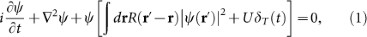with r = (x, y), 2 = ∂2/∂x2 + ∂2/∂y2, δT = Σn δ(tnT) a periodic delta-function of period T, R a general nonlinear response and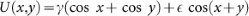a two dimensional periodic potential with strength defined byand γ. Equation (1) defines a two dimensional, nonlinear quantum kicked rotor: for R = 0 it reduces to the linear quantum kicked rotator22 while for U = 0 it corresponds to the 2D nonlinear Schrödinger equation (NLS), which represents a universal model of nonlinear waves in dispersive media30. In one dimension, conversely, Eq. (1) generalizes the nonlinear model investigated in27 with classical chaos parameter K = 2γT. Despite its deterministic nature, Eq. (1) can be precisely mapped to the Anderson model with a random potential11,32,33 and therefore furnishes a fundamental model for studying energy transport and dynamical localization34,35 in random systems.

This article is organized as follows. We begin by a series of experiments studying the diffusion of energy by integrating Eq. (1) in time with a parallel algorithm. We interpreted the results by an analytic theory based on Lie symmetry groups and soliton waves, which predicts a universal enhanced diffusion process, which is entirely sustained by nonlinearity. Analytical results are validated against numerical simulations, showing a perfect agreement with our predictions. We finally discuss the realization of a possible experiments in a multidimensional dipolar BEC, showing the feasibility of our ideas in a realistic setting.

## Results

### A first numerical experiment

We begin our analysis by calculating the momentum diffusionversus time, withthe momentum operator and 〈ψ|f|ψ〉 = ∫ drf|ψ|2 the quantum average. In order to investigate a general system, we considered a nonloncal diffusive nonlinear response n = ∫ drR(r′ − r)|ψ(r′)|2 following from: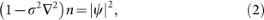with nonlocality controlled by σ. When σ = 0, the system response is local with n = |ψ|2. For σ ≠ 0, conversely, the system nonlinearity becomes long ranged with kernel given by, being K0 the modified Bessel function of second kind. Diffusive nonlinearities are particularly interesting in the context of nonlinear optics, as they can be easily accessed in liquids, as well as in Bose-Einstein Condensates (BEC), where they generalize previously investigated models36,37,38. Parallel numerical simulations are performed by a direct solution of (1) with an unconditionally stable algorithm. In order for the field ψ to explore the periodic potential U, we here consider wave packets whose spatial extension Δr 2π. Figure 1 summarizes our results obtained for σ = 0.2, by launching at the input a gaussian beamwith waist ω0 = 0.3 and amplitude A = 4 (Fig. 1a). The stochastic parameter K has been set to K = 1.8 > K*, above the stochastization threshold K* ≈ 0.97 where the linear classical uncoupled rotor exhibits diffusive transport in momentum space22. For comparison, we also calculated the linear dynamics resulting from R = 0 (Fig. 1b dotted line). As seen from Fig. 1b, the 2D nonlinear rotor behaves dramatically different with respect to its linear counterpart, demonstrating the strong role played by nonlinearity in the process. In particular, the linear system exhibits Anderson localization and diffusion suppression for(uncoupled condition), while for growingit shows a monotonically increasing sub-diffusion (Fig. 1b). In the nonlinear regime, conversely, Anderson localization is suppressed even forand the dynamics shows an erratic, random-like behavior that does not manifest any simple monotonic increase for growing values of. These results are also significantly different from the nonlinear kicked rotor in one dimension27, where nonlinearity was observed to induce Anderson localization effects thus suppressing any diffusive (or sub diffusive) regime.

### Analytic theory and Universal diffusion scaling

To theoretically investigate the results of Fig. 1 and derive predictions of universal character, we begin by calculating the time evolution of the wave packet center of mass, thus generalizing the Ehrenfest theorem of classical quantum mechanics: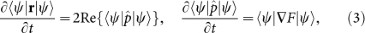with F = T + ∫ drR(rr′)|ψ(r′)|2. Equations (3) are valid for any dimensionality of the problem and for any nonlinearity R. In the following we assume a generic nonlinear response R that support at least a stable bound state of minimum energy, i.e., a nonlinear ground state solution. Numerical simulations performed in the previous section showed that the spatial field profile of the wavepacket, despite the chaotic motion, is not significantly altered in time (Fig. 1a,c). We can therefore describe the wavepacket dynamics in terms of a reduced set of coordinates modeling the nonlinear ground state of Eq. (1). To find the general form of the nonlinear ground state, we exploit Lie symmetry groups theory39. In particular, we start from the Lagrangian densityof Eq. (1), written for U = 0:and identify its variational symmetries, which we express by the following basis of Lie generators:These generators are associated to translational, rotational and Gauge symmetries of (1). The nonlinear ground state of (5) represents an invariant solutions with respect to the global symmetry group generated by: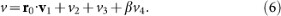We employed the method of characteristics39,40 to find the functional form of the general solution, which reads as follows: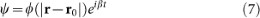with ϕ being a complex envelope. Equation (7) represents a soliton wave of the system. Closed form expression of ϕ for integrable responses R are found by the inverse scattering transform. In the case of Eq. (2), which possesses a nonintegrable response, we found approximate solutions by a variational analysis36,41. In particular, we use the following Gaussian ansatz: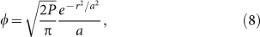defined by the power P = 〈ψ|ψ〉 and waist a(t). By substituting Eq. (8) in (4), after long but straightforward algebra we obtain the classical dynamics following from the Hamiltonian: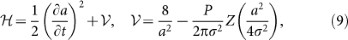with Z(x) = exΓ(0,x), Γ(0,x) the upper incomplete gamma function andthe potential of the one dimensional motion of a. The potentialhas a bell shape profile that possesses a unique absolute minimum V(a*) for every combination of P and σ. The fixed point a(0) = a* corresponds to a soliton wave of the system, which propagates in a translational fashion with fixed waist a(t) = a*, while different initial values lead to a breather42 characterized by a periodic oscillation of a in time. Figure 2a shows a typicalprofile obtained for P = 100 and different nonlocality degrees σ. As seen in the figure, the potential well becomes bigger and bigger for increasing non locality, explaining the dynamical robustness of the soliton dynamics during the chaotic motion observed in the parallel simulations performed in the previous section.

To investigate the motion of the soliton ground state in the general case when U ≠ 0 and R is arbitrary, we substitute Eq. (7) into (3) and perform an integration from nT to (n + 1)T. After some algebra, we end with the following system:with g = [sin x0, sin y0], u = [1, 1], classical position qnq(nT) and momentum pnp(nT) defined from q = 〈ψ|r0|ψ〉/〈ψ|ψ〉 and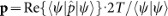, respectively. In the derivation of (10) we assumed the general condition of 〈ψ|(rr0)2|ψ〉 2π, in agreement with our introductory premises. Equations (10) represent a two dimensional standard map: for K > K*, above the stochastization threshold of the single uncoupled rotor, Eq. (10) is hyperchaotic and each dimension acts as an external noise source to the other, increasing the mixing of the overall system43. To highlight such a dynamics, we plot in Fig. 2b the positive Lyapunov exponent λ calculated for Eqs. (10). As seen, even when only a single rotor overcomes its stochastization threshold, it provides a noise source to the other giving rise to two positive Lyapunov exponents. The largest Lyapunov exponent grows linearly with(Fig. 2b). The hyperchaotic nature of Eq. (10) is expect to strongly affect the momentum diffusion in the phase space. We investigate the latter by generalizing the approach of Rechester and White developed for turbulent flows44. The classical diffusion D of the map can be expressed as follows:being Δp = pnp0 andthe probability distribution of position pn and momentum qn at time n (measured in kick units). To calculate, we begin by observing that the the evolution of the map (10) at the n-th kick can be written as follows: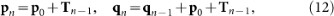expressed as a function of the initial momentum p0, with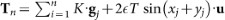and gj = [sin xj, sin yj]. The evolution of the probability densityat time n is then given by:being(q0, p0,0) the initial density. The presence of the additional summations over h originates from the periodicity of the position q in the phase space. By considering a general uniform initial distribution with all the particles possessing nonzero momentum p, i.e.,, after substituting into (13) and (15), we obtain: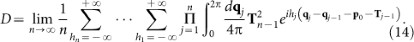The leading order in the integral is obtained by neglecting any folding in the position space, i.e., by assuming hj = 0 (for hj ≠ 0, conversely, we get asymptotically small corrections expressed in terms of Bessel functions that we do not reported here due to their cumbersome expressions). The leading diffusion reads as follows: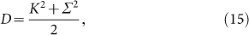being. Equation (15) is to be considered of universal applicability, i.e., for any system dimension and for arbitrary nonlinear responses, as it has been derived under the general equations (3), (10)–(15).

## Discussion

Equation (15) allows to fully interpret the nonlinear dynamics of Eq. (1). In particular, the quantum average 〈P〉 results from an hyperchaotic system described by a two dimensional dimensional standard map and each realization manifests itself as a random walk in Fig. 1b. The map diffusion rate is identical to the momentum diffusion of the classical linear rotor22, hence, an additional average (in time or over an ensemble of input conditions) re-establishes a perfect classical correspondence for every coupling. It is worthwhile observing that the classical correspondence in the multidimensional linear quantum rotor is manifested only for very high couplingand in general the quantum diffusion 〈P〉 follows a fractional behavior with 〈P tβ<1 (see e.g.22, or Fig. 1b dashed lines). As a result, the linear quantum rotor sub-diffuses at a slower rate than its classical counterpart. Conversely, Eq. (15) predicts a perfect classical correspondence for every coupling, which is re-established thanks to nonlinear effects. In order to verify Eq. (15) and to demonstrate such a nonlinearly-enhanced transport dynamics, we performed extensive numerical simulations from Eq. (1) and calculated the average diffusion through a quantum average followed by an average over different input conditionsFigure 3 summarizes our results obtained for K = 5, σ = 0.2 and by considering an initial wave packet composed by a Gaussian beam with waist ω0 = 0.3 and amplitude A = 4. In complete agreement with Eqs. (10)–(15), we observe a diffusive behaviorfor every(Fig. 3a solid lines), whose rate is exactly matching our theoretical prediction based on Eq. (15) [Fig. 3a dashed lines]. Figure 3b compares linear and nonlinear evolution of Eq. (1), the former obtained for R = 0. As seen in the figure, the nonlinear enhancement in the diffusion due to the restoration of classical effect is significant and it increases with the coupling. This can be intuitively expected due to the fact that an increasing coupling leads to an increasing mixing in the system and to stronger diffusion effects. The relative diffusion variation, normalized respect to the nonlinear dynamics, is approximatively 50% at, which means that nonlinearity is significantly faster (by a factor of two) in transporting energy.

In order to discuss a possible experimental realization and further verify the universality of our predictions, we considered the case of a multidimensional dipolar BEC41. This system attracted a conspicuous interest in the scientific community due to its important implications in many-body dynamics, quantum computing and nonlinear waves28,45,46,47,48. Dipolar BEC are characterized by long range interactions, which support stable ground state solitons and high order azimutons28,48. The nonlinear dynamics of the wavefunction in a kicked optical lattice can be written in the following adimensional form: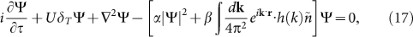with dimensionless τ = z,,,,,,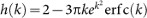, where,is the Fourier transform of |Ψ|2, ωz is the BEC trapping frequency along z,, g the coupling constant characterizing two-body contact interactions, gd the strength of the dipole-dipole interaction potential28 and ρ a reference BEC density. Interaction terms g and gd can be tuned via Feshbach resonance, which allows to experimentally control the ratio gd/g that determines the properties of the nonlinear ground state of the condensate. In our numerical experiments, we considered gd/g = −0.5, as a feasible value for the generation of a stable two-dimensional soliton state28.

Figure 4 summarizes our results for K = 2, T = 1 and different coupling strengths. In perfect agreement with our theory, the averaged momentum diffuses as predicted by our universal formula, increasing its strength as the couplinggrows. The final density distribution, after τ = 50T, shows the stable propagation of the dipolar ground state that is evolving inside the system (Fig. 4b), proving the feasibility of our predictions in a realistic system.

In conclusion, motivated by the large interest in the study of the energy transport in disordered system, we investigated the role of nonlinearity in affecting the dynamics of energy diffusion in random media. This problem has also profound implications in quantum chaos and time reversibility of classical systems, where nonlinearity emerges naturally when we consider interacting particles. We considered a universal model of wave propagation, namely the multidimensional quantum kicked rotor in presence of a generic atom-atom interactions. We began our analysis with a single numerical experiment and studied the behavior of the energy diffusion in the phase space, finding unexpected results that are very different with respect to both linear multidimensional dynamics and nonlinear evolutions of one dimensional systems. We tackled the problem by employing a combination of soliton theory and Lie symmetry analysis, finding a universal diffusion evolution of the energy that follows from the full restoration of classical effects sustained by soliton waves. The latter, in particular, breaks Anderson localization effects and diffuse energy with a larger rate with respect to linear systems of the same size. Numerical simulations performed on different models, including dipolar BEC, perfectly agree with our universal predictions. From a pure quantum perspective, our results demonstrate that atom-atom interactions inhibit quantum time reversal in many dimensions, due to the full recovery of classical chaotic mixing in the system. This generalizes the intuition of Adachi, Today and Ikeda22, who found that in linear regime multidimensional quantum time reversal is only conditionally possible, in the sense that anthreshold exists for the recovery of the initial wavepacket, while beyond a specific interactionno reversal is possible. When nonlinearity is taken into account, conversely, solitons reestablish a fully mixing dynamics and for all values ofno time reversal can be observed.

We can therefore conclude that nonlinear waves can favor the energy transport in a disordered medium and significantly speed up the process of energy diffusion when compared to linear dynamics. Solitons can be therefore used for the development of new architectures for enhancing the transport of matter in disordered materials.

## Methods

Numerical simulations of Eq. (1) have been realized by an homemade parallel code based on an unconditionally stable, second order time marching scheme. Parallelization is achieved by a two dimensional domain decomposition strategy, where each part of the computational domain is assigned to a different processor, with all communications written following the MPI standard. The numerical results presented in this work have been realized by 200000 single cpu hours on 128 processors of our “reddragon” linux cluster.

## References

1. Sheng, P. Introduction to Wave Scattering, Localization and Mesoscopic Phenomena. (Academic Press, San Diego, 1995).

2. Scheffold, F., Lenke, R., Tweer, R. & Maret, G. Localization or classical diffusion of light. Nature 398, 206 (1999).

3. Schwartz, T., Bartal, G., Fishman, S. & Segev, M. Transport and anderson localization in disordered two-dimensional photonic lattices. Nature 446, 52 (2007).

4. Skipetrov, S. E. & van Tiggelen, B. A. Dynamics of anderson localization in open 3d media. Phys. Rev. Lett. 96, 043902 (2006).

5. Conti, C. & Fratalocchi, A. Dynamic light diffusion, three-dimensional anderson localization and lasing in inverted opals. Nature Phys. 4, 794 (2008).

6. Lee, P. A. & Ramakrishnan, T. V. Disordered electronic systems. Rev. Mod. Phys. 57, 287–337 (1985).

7. Lahini, Y. et al. Anderson localization and nonlinearity in one-dimensional disordered photonic lattices. Phys. Rev. Lett. 100, 013906 (2008).

8. Billy, J. et al. Direct observation of anderson localization of matter waves in a controlled disorder. Nature 453, 891 (2008).

9. Roati, G. et al. Anderson localization of a non-interacting bose-einstein condensate. Nature 453, 895 (2008).

10. Efetov, K. Supersymmetry in disorder and chaos (Cambridge, New York, 1997).

11. Fishman, S., Grempel, D. R. & Prange, R. E. Chaos, quantum recurrences and anderson localization. Phys. Rev. Lett. 49, 509–512 (1982).

12. Molinari, D. & Fratalocchi, A. Route to strong localization of light: the role of disorder. Opt. Express 20, 18156–18164 (2012).

13. Liu, C. et al. Enhanced energy storage in chaotic optical resonators. Nat. Photon 7, 473–478 (2013).

14. Mayou, D., Berger, C., Cyrot-Lackmann, F., Klein, T. & Lanco, P. Evidence for unconventional electronic transport in quasicrystals. Phys. Rev. Lett. 70, 3915–3918 (1993).

15. Levi, L. et al. Disorder-enhanced transport in photonic quasicrystals. Science 332, 1541 (2011).

16. Madhukar, A. & Post, W. Exact solution for the diffusion of a particle in a medium with site diagonal and off-diagonal dynamic disorder. Phys. Rev. Lett. 39, 1424–1427 (1977).

17. Izrailev, F. M. & Krokhin, A. A. Localization and the mobility edge in one-dimensional potentials with correlated disorder. Phys. Rev. Lett. 82, 4062–4065 (1999).

18. Levi, L., Krivolapov, Y., Fishman, S. & Segev, M. Hyper-transport of light and stochastic acceleration by evolving disorder. Nat. Phys. 8, 912–917 (2012).

19. Loschmidt, J. Sitzungsber. kais. akad. wiss. wien. Math. Naturwiss. Classe 73, 128 (1876).

20. Driebe, D. J. Fully Chaotic Maps and Broken Time Symmetry (Kluwer, Norwell, 1999).

21. Martin, J., Georgeot, B. & Shepelyansky, D. L. Time reversal of bose-einstein condensates. Phys. Rev. Lett. 101, 074102 (2008).

22. Adachi, S., Toda, M. & Ikeda, K. Quantum-classical correspondence in many-dimensional quantum chaos. Phys. Rev. Lett. 61, 659–661 (1988).

23. Gaspard, P. et al. Experimental evidence for microscopic chaos. Nature 394, 865 (1998).

24. Cencini, M., Falcioni, M., Olbrich, E., Kantz, H. & Vulpiani, A. Chaos or noise: Difficulties of a distinction. Phys. Rev. E 62, 427–437 (2000).

25. Maccone, L. Quantum solution to the arrow-of-time dilemma. Phys. Rev. Lett. 103, 080401 (2009).

26. Anastopoulos, C. & Savvidou, N. Consistent thermodynamics for spin echoes. Phys. Rev. E 83, 021118 (2011).

27. Benvenuto, F., Casati, G., Pikovsky, A. S. & Shepelyansky, D. L. Manifestations of classical and quantum chaos in nonlinear wave propagation. Phys. Rev. A 44, R3423–R3426 (1991).

28. Griesmaier, A., Werner, J., Hensler, S., Stuhler, J. & Pfau, T. Bose-einstein condensation of chromium. Phys. Rev. Lett. 94, 160401 (2005).

29. Klawunn, M., Nath, R., Pedri, P. & Santos, L. Transverse instability of straight vortex lines in dipolar bose-einstein condensates. Phys. Rev. Lett. 100, 240403 (2008).

30. Griffin, A., Snoke, D. W. & Stringari, S. Bose-Einstein Condensation (Cambridge University Press, Cambridge, 1995).

31. Ivanchenko, M. V., Laptyeva, T. V. & Flach, S. Anderson localization or nonlinear waves: A matter of probability. Phys. Rev. Lett. 107, 240602 (2011).

32. Haake, F. Quantum Signatures of Chaos (Springer, New York, 2001).

33. Grempel, D. R., Prange, R. E. & Fishman, S. Quantum dynamics of a nonintegrable system. Phys. Rev. A 29, 1639–1647 (1984).

34. Buchleitner, A. & Delande, D. Dynamical localization in more than one dimension. Phys. Rev. Lett. 70, 33–36 (1993).

35. Casati, G., Chirikov, B. V., Shepelyansky, D. L. & Guarneri, I. Relevance of classical chaos in quantum mechanics: The hydrogen atom in a monochromatic field. Physics Reports 154, 77–123 (1987).

36. Bang, O., Krolikowski, W., Wyller, J. & Rasmussen, J. J. Collapse arrest and soliton stabilization in nonlocal nonlinear media. Phys. Rev. E 66, 046619 (2002).

37. Fratalocchi, A., Conti, C., Ruocco, G. & Trillo, S. Free-energy transition in a gas of noninteracting nonlinear wave particles. Phys. Rev. Lett. 101, 044101 (2008).

38. Greeff, C. W. Molecular orientational relaxation and the thermal nonlinear refraction of nematic liquid crystals. Molecular Crystals and Liquid Crystals Science and Technology. Section A. Molecular Crystals and Liquid Crystals 238, 179–195 (1994).

39. Olver, P. J. Applications of Lie groups to differential equations (Springer-Verlag, New York, 1986).

40. Bluman, G. W. & Anco, S. C. Symmetries and integration methods for Differential Equations (Springer, New York, 2002).

41. Pedri, P. & Santos, L. Two-dimensional bright solitons in dipolar bose-einstein condensates. Phys. Rev. Lett. 95, 200404 (2005).

42. Snyder, A. W. & Mitchell, D. J. Accessible solitons. Science 276, 1538–1541 (1997).

43. Adachi, S., Toda, M. & Ikeda, K. Potential for mixing in quantum chaos. Phys. Rev. Lett. 61, 655–658 (1988).

44. Rechester, A. B. & White, R. B. Calculation of turbulent diffusion for the chirikov-taylor model. Phys. Rev. Lett. 44, 1586–1589 (1980).

45. Góral, K., Santos, L. & Lewenstein, M. Quantum phases of dipolar bosons in optical lattices. Phys. Rev. Lett. 88, 170406 (2002).

46. Baranov, M. A., Osterloh, K. & Lewenstein, M. Fractional quantum hall states in ultracold rapidly rotating dipolar fermi gases. Phys. Rev. Lett. 94, 070404 (2005).

47. DeMille, D. Quantum computation with trapped polar molecules. Phys. Rev. Lett. 88, 067901 (2002).

48. Skupin, S., Bang, O., Edmundson, D. & Krolikowski, W. Stability of two-dimensional spatial solitons in nonlocal nonlinear media. Phys. Rev. E 73, 066603 (2006).

## Acknowledgements

A. Fratalocchi acknowledges funding from KAUST (Award No. CRG-1-2012-FRA-005 and Award No. GRP-CF-2011-09-P). For parallel computing, this research used the resources of the Primalight group at KAUST University.

## Author information

Authors

### Contributions

A.F. conceived the work and developed the theoretical analysis. D.S.B. performed the numerical simulations. A.F. wrote the manuscript. All authors reviewed the manuscript.

## Ethics declarations

### Competing interests

The authors declare no competing financial interests.

## Rights and permissions

Reprints and Permissions

Brambila, D., Fratalocchi, A. Nonlinearly-enhanced energy transport in many dimensional quantum chaos. Sci Rep 3, 2359 (2013). https://doi.org/10.1038/srep02359

• Accepted:

• Published: